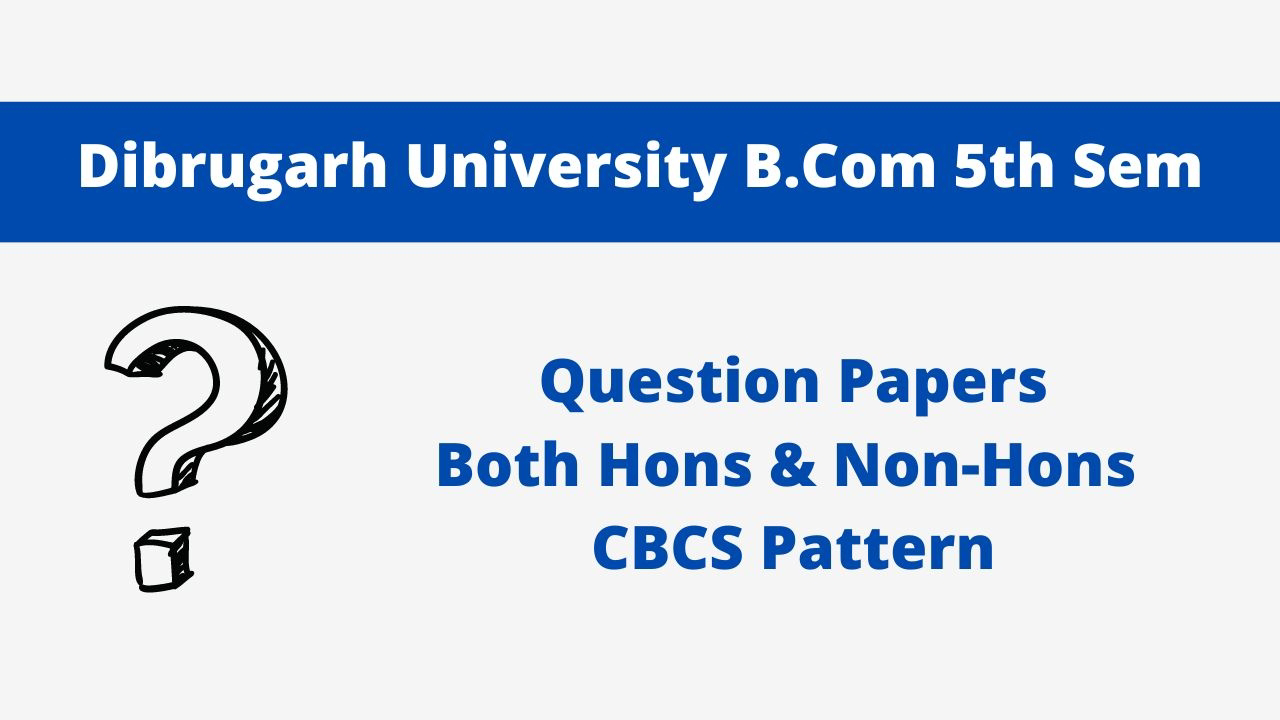# Principles of Microeconomics Question Paper 2021, Dibrugarh University B.Com 5th Sem CBCS Pattern

## Dibrugarh University B. Com 5th Sem Question Papers CBCS Pattern5th SEM TDC PM (CBCS) GE 501Principles of Microeconomics 2021(Held in January/February, 2022)COMMERCE (Generic Elective)Paper: GE 501 (Principles of Microeconomics)Full Marks: 80

Pass Marks: 32

Time: 3 hours.

The figures in the margin indicate full marks for the questions.

1. Answer the following as directed:                                   1x8=8

(a)  The demand for a commodity is inversely related to the price of its substitutes. (Write True or False)

(b) If demand curve is parallel to Y-axis, the price elasticity of demand is equal to unity/more than unity/less than unity/zero. (Choose the correct answer)

(c)  What is ordinal measurement of utility?

(d) Define a price consumption curve.

(e)  Total cost is the summation of (Choose the correct answer)

(1)  Total fixed cost and total variable cost.

(2)  Average cost and marginal cost.

(3)  Selling cost and money cost.

(4)  Real cost and opportunity cost.

(f)   If two factors are perfectly substitutes, the isoquant curve will be (Choose the correct answer)

(1)  Negatively sloping convex curve.

(2)  Negatively sloping straight line.

(3)  Right-angled.

(4)  None of the above.

(g)  Under which form of market a firm is price taker?  (Choose the correct answer)

(1)  Monopoly.

(2)  Perfect competition.

(3)  Monopolistic competition.

(4)  Oligopoly.

(h) Monopolistic competition and oligopoly are alike in terms of _______. (Fill in the blank)

2. Write short notes on any four of the following:              4x4=16

(a)  Determinants of price elasticity of supply.

(b) Characteristics of indifference curve.

(c)  Relationship between average cost and marginal cost.

(e)  Excess capacity in monopolistic competition.

3. (a) Explain the relationship price of a commodity and its quantity supplied. Discuss how market equilibrium is determined at the intersection of market demand and market supply curves.       4+7=11

Or

(b) What is price elasticity of demand? Examine the role of price elasticity of demand in decision making of a firm. 3+8=11

4. (a) What is consumer’s equilibrium? Explain how a consumer attains equilibrium with the indifference curve and budget line.        4+8=12

Or

(b) Define income effect and substitution effect. Explain how price effect of a commodity is decomposed into income effect and substitution effect.    4+8=12

Principles of Microeconomics Solved Question Paper 2021 (B.Com 5th Sem)

5. (a) Discuss the law of variable proportions using an appropriate production. At which stage, the producer stops his/her production?        9+2=11

Or

(b) What are economies of scale? Distinguish between the internal and external economies of scale.         2+9=11

6. (a) Discuss the main features of a perfectly competitive market. Explain how a firm under perfect competition attains equilibrium with normal profit, super-normal profit and loss in the short run.       4+7=11

Or

(b) What is price discrimination? Discuss the type of price discrimination with examples. Discuss the conditions of price discrimination. 2+6+3=11

7. (a) Define monopolistic competition. Discuss the price-output determination under monopolistic competition both in short and long run.     2+9=11

Or

(b) What is oligopoly? What are its characteristics? What factors cause the emergence of oligopoly?          2+3+6=11

***